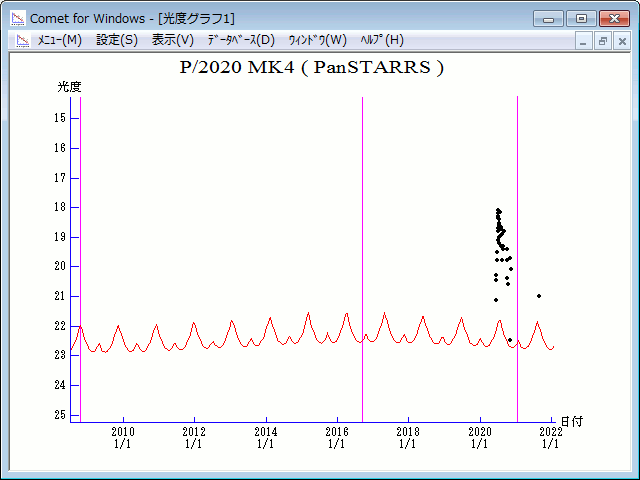# \$B%Q%s%9%?!<%:WB@1(B

P/2020 MK4 ( PanSTARRS )###\$B%W%m%U%#!<%k(B

 \$BH/8+F|(B 2020\$BG/(B6\$B7n(B24\$BF|(B \$BH/8+8wEY(B 19.8\$BEy(B \$BH/8+ Pan-STARRS 1 telescope (Haleakala)

###\$B50F;MWAG(B

```                    Epoch = 2016 Sept. 9.0 TT
T = 2016 Sept.18.30133 TT        Peri. = 199.54417
e = 0.0433682                    Node  =   4.96322 2000.0
q = 6.0390160 AU                 Incl. =   6.69876
a =  6.3127902 AU   n = 0.06214011   P =  15.86 years
```

###\$B8wEYJQ2=(B

```        H = 14.0  G = 0.15
```##### \$B50F;MWAG\$O!"(BCBET 5191\$B\$K7G:\\$5\$l\$?\$b\$N\$G\$9!#(B \$B8wEY%0%i%U\$O(BComet for Windows\$B\$G:n@.\$7\$?\$b\$N\$G\$9!#(B